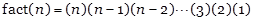How to Enter Answers in WeBWorK

 Addition + a+b givesSubtraction - a-b gives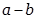Multiplication * a*b givesMultiplication may also be indicated by a space or juxtaposition, such as 2x, 2 x, 2*x, or 2(x+y). Division / a/b givesExponents ^ or ** a^b gives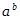as does a**b Parentheses, brackets, etc (...), [...], {...}

Syntax for entering expressions

·         Be careful entering expressions just as you would be careful entering expressions in a calculator.

·         Sometimes using the * symbol to indicate multiplication makes things easier to read. For example (1+2)*(3+4) and (1+2)(3+4) are both valid. So are 3*4 and 3 4 (3 space 4, not 34) but using an explicit multiplication symbol makes things clearer.

·         Use parentheses (), brackets [], and curly braces {} to make your meaning clear.

·         Do not enter 2/4+5 (which is 5 ½ ) when you really want 2/(4+5) (which is 2/9).

·         Do not enter 2/3*4 (which is 8/3) when you really want 2/(3*4) (which is 2/12).

·         Entering big quotients with square brackets, e.g. [1+2+3+4]/[5+6+7+8], is a good practice.

·         Be careful when entering functions. It is always good practice to use parentheses when entering functions. Write sin(t) instead of sint or sin t. WeBWorK has been programmed to accept sin t or even sint to mean sin(t). But sin 2t is really sin(2)t, i.e. (sin(2))*t. Be careful.

·         Be careful entering powers of trigonometric, and other, functions. You write (sin(t))^2 for the square of sin(t), and never sin^2t.

·         For example for the expression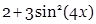, 2+3sin^2(4x) is wrong. You should enter: 2+3*(sin(4*x))^2. Why does the last expression work?

Please Excuse My Dear Aunt Sally

Operations in parentheses are always done first (4*x) and then (sin(4*x))], next all exponents are taken, giving (sin(4*x))^2, next all multiplications and divisions are performed, giving 3*(sin(4*x))^2. Finally, all additions and subtractions are performed, giving 2+3*(sin(4*x))^2.

·         Remember that multiplication and division have the same precedence and there are no universal rules as to which should be done first in the absence of parentheses.  WeBWorK and many computers read things from left to right, so 2/3*4 means (2/3)*4=8/3. But some other computers will read 2/3*4 as 2/(3*4)=1/6.  The same lack of consistent rules concerns powers, expressions like 2^3^4.

The only way to insure that you are entering what you want to enter is the use of parentheses!!!

·         Use the Preview Button to see exactly how your entry appears to the system. For example, to tell the difference between 1+2/3+4 and [1+2]/[3+4] click the Preview Button.

·         If a problem calls for a decimal answer, give at least four decimal digits, or as many as the problem specifies. For example, write 2.3453 instead of 2.34.

Intervals in WeBWorK

What is the domain of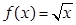? One answer is x>=0 (x is greater than or equal to 0). The best way to enter this in WeBWorK is by using interval notation: [0,infinity).

Other intervals:

(2,3] is the set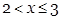.

(-infinity,5) is the set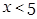.

(-infinity, infinity) is the set of all real numbers.

(2,3]U[4,5) is the set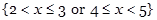. (This is a union of two intervals and can be very important.)

Mathematical Constants Available In WeBWorK

pi   This gives π ≈ 3.14159265358979. So cos(pi) is –1.

e    This gives e ≈ 2.718281828459045. So, ln(e*2) is 1 + ln(2)

Scientific Notation Available In WeBWorK

2.1E2 gives 210

2.1E-2 gives 0.021

aEb gives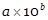Cube roots and nth Roots

x^(1/3) gives, the cube root of x

x^(1/n) gives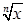, the nth root of x

x^(p/q) givesMathematical Functions Available In WeBWorK

abs( )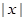, the absolute value

cos( )        the cosine function.  Note: the cosine function uses radian measure

sin( )         the sine function.  Note: the sine function uses radian measure

tan( )        the tangent function.  Note: the tangent function uses radian measure

sec( )        the secant function.  Note: the secant function uses radian measure and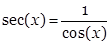exp( )        the exponential function, ex

log( )        The natural logarithm function.  Note that this is NOT the common log function from pre-calculus.

ln( )           Another, more common name for the natural logarithm,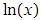logten( )   The common logarithm or log base 10,arcsin( )    The inverse sine function.  asin( ) is another name for arcsine.

arccos( )  The inverse cosine function.  acos( ) is another name for arccosine.

arctan( )  The inverse tangent function.  atan( ) is another name for arctangent.

sqrt( )     The square root function

sgn( )        The sign function —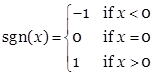step( )     The step function —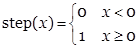(0 if x < 0, 1 if x >= 0)

fact( )       The factorial function (defined only for non-negative integers),